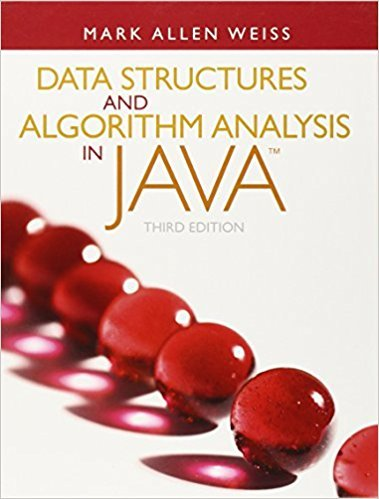×
Get Full Access to Data Structures And Algorithm Analysis In Java - 3 Edition - Chapter 9 - Problem 9.4
Get Full Access to Data Structures And Algorithm Analysis In Java - 3 Edition - Chapter 9 - Problem 9.4

×

# An adjacency matrix requires O(|V|2) merely to initialize using a standard doubleloopISBN: 9780132576277 316

## Solution for problem 9.4 Chapter 9

Data Structures and Algorithm Analysis in Java | 3rd Edition

• Textbook Solutions
• 2901 Step-by-step solutions solved by professors and subject experts
• Get 24/7 help from StudySoup virtual teaching assistantsData Structures and Algorithm Analysis in Java | 3rd Edition

4 5 1 313 Reviews
12
4
Problem 9.4

An adjacency matrix requires O(|V|2) merely to initialize using a standard doubleloop. Propose a method that stores a graph in an adjacency matrix (so that testingfor the existence of an edge is O(1)) but avoids the quadratic running time.

Step-by-Step Solution:
Step 1 of 3

BSC 103 Week 5 Playing With DNA to do handy things  Dna contains the instruction manual to make the proteins your body needs  Every structure has a unique sequence  Many many applications o Crime scenes o First used to solve a crime in 1986  Present in almost every cell in your body  How we go from evidence to a solved case o Need a sample that has the suspect’s DNA o Extract samples from the DNA o Amplify using the Polymerase chain reaction  A laboratory technique that replicates a certain DNA The Polymerase Chain Reaction  Laboratory technique used to replicate and amplify a specific DNA segment  Primers: short segments of DNA that guide DNA polymerase to the section of DNA to copy  3 main steps o Heat to separate the strands o Anneal primers o Elongate strands  Allows dna replication to occur many times  Can make billions of copies from a starting sample or just a few molecules of DNA After Pcr, we create DNA profiles  Dna take profiling adavantage of the fact that no two people have the exact same DNA sequence or genome…each person has a unique DNA” fingerprint” o Genome: One complete set of genetic instructions encoded in the DNA of an organism DNA profiling  we could sequence the whole genome from cells found at the scene, as well as from suspects.  Shortcut o Use prc to amplify only specific segments

Step 2 of 3

Step 3 of 3

## Discover and learn what students are asking

Calculus: Early Transcendental Functions : Differential Equations: Growth and Decay
?In Exercises 1-10, solve the differential equation. $$\frac{d y}{d x}=5-8 x$$

Calculus: Early Transcendental Functions : First-Order Linear Differential Equations
?In Exercises 5-14, solve the first-order linear differential equation. $$\frac{d y}{d x}+\left(\frac{1}{x}\right) y=6 x+2$$

Calculus: Early Transcendental Functions : Integration by Tables and Other Integration Techniques
?In Exercises 47-52, verify the integration formula. \(\int \frac{u^{n}}{\sqrt{a+b u}} d u=\frac{2}{(2 n+1) b}\left(u^{n} \sqrt{a+b u}-n a \in

Statistics: Informed Decisions Using Data : Testing the Significance of the Least-Squares Regression Model
?If H0: b1 = 0 is not rejected, what is the best estimate for the value of the response variable for any value of the explanatory variable?

Unlock Textbook Solution